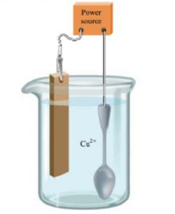# Copper can be plated onto a spoon by placing the spoon in an acidic solution of CuSO 4 ( aq ) and connecting it m a copper strip via a power source as illustrated below: a. Label the anode and cathode. and describe the direction of the electron now. b. Write out the chemical equations for the reactions that occur at each electrode.### Chemistry: An Atoms First Approach

2nd Edition
Steven S. Zumdahl + 1 other
Publisher: Cengage Learning
ISBN: 9781305079243

#### Solutions

Chapter
Section### Chemistry: An Atoms First Approach

2nd Edition
Steven S. Zumdahl + 1 other
Publisher: Cengage Learning
ISBN: 9781305079243
Chapter 17, Problem 108E
Textbook Problem
9 views

## Copper can be plated onto a spoon by placing the spoon in an acidic solution of CuSO4(aq) and connecting it m a copper strip via a power source as illustrated below:a. Label the anode and cathode. and describe the direction of the electron now.b. Write out the chemical equations for the reactions that occur at each electrode.

(a)

Interpretation Introduction

Interpretation:

The set up of a cell in which Copper can be plated onto a spoon by placing it in an acidic solution of CuSO4(aq) and its connection to a Copper strip via power source is given. The labeling of the anode and cathode in the given set up and the direction of electron flow is to be stated.

Concept introduction:

The non-spontaneous reaction takes place in an electrolytic cell in which there occurs conversion of electrical energy into chemical energy and this is used for the electrolysis of a metal.

The solution of CuSO4(s) contains Cu2+ and SO42 ions. When a current is passed through the solution, the Cu2+ ions accept electrons from the cathode. After accepting the electrons, the Cu2+ ions get reduced and get deposited at the spoon. As the reduction reaction takes place over the cathode, so the spoon acts as the cathode, while the Copper strip acts as the anode.

To determine: The labeling of the anode and cathode in the given set up and the direction of electron flow.

### Explanation of Solution

Explanation

The spoon acts as the cathode, while the Copper strip acts as the anode and the transfer of electrons occur from anode to cathode.

The direction of electron flow is shown as,

(b)

Interpretation Introduction

Interpretation:

The set up of a cell in which Copper can be plated onto a spoon by placing it in an acidic solution of CuSO4(aq) and its connection to a Copper strip via power source is given. The labeling of the anode and cathode in the given set up and the direction of electron flow is to be stated.

Concept introduction:

The non-spontaneous reaction takes place in an electrolytic cell in which there occurs conversion of electrical energy into chemical energy and this is used for the electrolysis of a metal.

The solution of CuSO4(s) contains Cu2+ and SO42 ions. When a current is passed through the solution, the Cu2+ ions accept electrons from the cathode. After accepting the electrons, the Cu2+ ions get reduced and get deposited at the spoon. As the reduction reaction takes place over the cathode, so the spoon acts as the cathode, while the Copper strip acts as the anode.

To determine: The chemical equations for the reactions that occur at each electrode.

The chemical reactions taking place at the anode is,

CuCu2++2e

The chemical reaction taking place at cathode is,

Cu2++2eCu

### Still sussing out bartleby?

Check out a sample textbook solution.

See a sample solution

#### The Solution to Your Study Problems

Bartleby provides explanations to thousands of textbook problems written by our experts, many with advanced degrees!

Get Started

Find more solutions based on key concepts
For managing type 2 diabetes, regular physical activity can help by redistributing the body's fluids. T F

Nutrition: Concepts and Controversies - Standalone book (MindTap Course List)

1.71 (Chemical Connections 1B) How does the body react to hypothermia?

Introduction to General, Organic and Biochemistry

Section 4.5 Tangential and Radial Acceleration Figure P4.40 represents the total acceleration of a particle mov...

Physics for Scientists and Engineers, Technology Update (No access codes included)

Is a tsunami a shallow-water wave or a deep-water wave?

Oceanography: An Invitation To Marine Science, Loose-leaf Versin